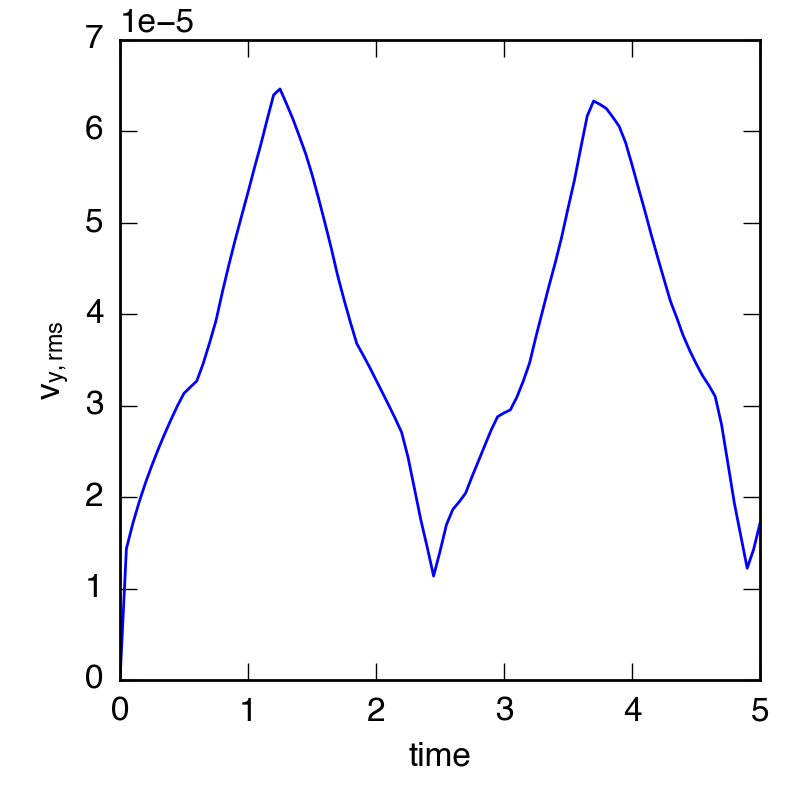# Gravity (Part 2)

## December 14, 2016

In addition to the operator-split source term update I added last week,

$$(\rho v)^{n+1}_{i} = (\rho v)^{n}_{i} + \frac{\Delta t}{2} g (\rho^{n}_{i} + \rho^{n+1}_{i}),$$ $$(\rho E)^{n+1}_{i} = (\rho E)^{n}_{i} + \frac{\Delta t}{4} g (\rho^{n}_{i} + \rho^{n+1}_{i})(v^{n}_{i} + v^{n+1}_{i}),$$

I’ve now tried correcting the velocities and total energy before the input states are passed to the Riemann solver. The correction takes the same form as the source terms, so for example, the correction to the left states would be:

$$(\rho_L v_{x_L}) += \Delta t \rho_{L} g_{x}$$ $$(\rho_L v_{y_L}) += \Delta t \rho_{L} g_{y}$$ $$(\rho_L v_{z_L}) += \Delta t \rho_{L} g_{z}$$ $$(\rho_L E_{L}) += \Delta t \rho_{L} (v_{x, L} g_x + v_{y, L} g_y + v_{z, L} g_z),$$

where $g_x$, $g_y$, and $g_z$ are the three components of the gravitational acceleration.

I’ve tested the two versions (the original update versus the corrected input states) using the same Rayleigh-Taylor initial conditions as last week’s post, but without the velocity perturbation. Because the fluid is in hydrostatic equilibrium to start, the fluctuations in the rms y-velocities seem like a decent measure of how well the code is doing. I found (somewhat surprisingly) that there is virtually no difference between the two methods. The plot below shows the rms velocities for the original version - the corrected version looks exactly the same. All tests so far have been run using PPMC, an exact Riemann solver, and CTU.